Next: Bibliography Up: Calculus of Variations Previous: Multi-Function Variation

# Exercises

1. Find the extremal curves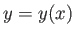of the following constrained optimization problems, using the method of Lagrange multipliers:
1.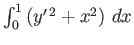, such that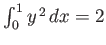.
2.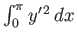, such that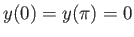, and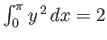.
3.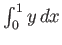, such that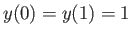, and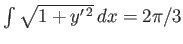.
2. Suppose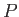and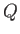are two points lying in the-plane, which is orientated vertically such thatis above. Imagine there is a thin, flexible wire connecting the two points and lying entirely in the-plane. A frictionless bead travels down the wire, impelled by gravity alone. Show that the shape of the wire that results in the bead reaching the pointin the least amount of time is a cycloid, which takes the parametric form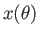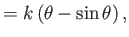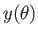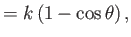where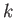is a constant.
3. Find the curve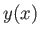, in the interval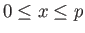, which is of length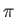, and maximizes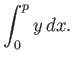Next: Bibliography Up: Calculus of Variations Previous: Multi-Function Variation
Richard Fitzpatrick 2016-03-31